• 十进制转二进制class DecToBin{public static void main(String[] args){//System.out.println("Hello World!");long dec = -9223372036854775807l;// -9223372036854775808 这个数不行，不要试，嘿嘿String binStr=...

十进制转二进制

class DecToBin

{

public static void main(String[] args)

{

//System.out.println("Hello World!");

long dec = -9223372036854775807l;

// -9223372036854775808 这个数不行，不要试，嘿嘿

String binStr="";

long decAbs=Math.abs(dec);

while (decAbs>0)

{ binStr=(decAbs&1)+binStr;

decAbs>>=1;

}

binStr= dec<0?"-"+binStr:dec==0?"0":binStr;

System.out.println(binStr);

}

}

十进制转八进制

class DecToOct

{

public static void main(String[] args)

{

//System.out.println("Hello World!");

long dec=-0;//有-0 吗？

String octStr="";

long decAbs=Math.abs(dec);

while (decAbs>0)

{ octStr=(decAbs&7)+octStr;//

decAbs>>=3;

}

octStr= dec<0?"-"+octStr:dec==0?"0":octStr;

System.out.println(octStr);

}

}

十进制转十六进制

class DecToHex

{

public static void main(String[] args)

{

System.out.println("Hello World!");

long dec=-1;//计算器的负数不会弄 -。-

String hexStr="";

long decAbs=Math.abs(dec);

while(decAbs>0)

{ long lastFour=decAbs&15;

if (lastFour>9)

hexStr=(char)('A'+lastFour-10)+hexStr;

else hexStr=lastFour+hexStr;

decAbs>>=4;

}

hexStr= dec<0?"-"+hexStr:dec==0?"0":hexStr;

System.out.println(hexStr);

}

}

以上就是本文的全部内容，希望本文的内容对大家的学习或者工作能带来一定的帮助，同时也希望多多支持聚米学院！

展开全文• 1、不使用字符串操作函数，把一个十进制字符串转换成二进制字符串 函数原型： char* Dec2Bin(const char* dec, char* bin, int size) { } 调用： { char szBuff; const char* test = "0096"; cout (test, ...

1、不使用字符串操作函数，把一个十进制字符串转换成二进制字符串

函数原型：

char* Dec2Bin(const char* dec, char* bin, int size)
{
}

调用：

{
char szBuff;
const char* test = "0096";
cout << Dec2Bin(test, szBuff, 33);
}

具体实现：

//把原始字符串转换成整数
int myatoi(const char* dec)
{
int num = 0;
while (*dec != '\0')
{
if (*dec >= '0' && *dec <= '9')
{
num = num * 10 + (*dec - '0');
}
else     //防止非法字符串（非数字）
{
num = 0;
break;
}
dec++;
}

return num;
}

//把整数转换成二进制字符串
char* myitoa(int num, char *str, int size)
{
char *temp = new char[size];
int a, i = 0, j = 0;

while (num > 0)
{
a = num % 2;
temp[j++] = a + '0';      //求余

num /= 2;
}

while (j > 0)
{
str[i++] = temp[--j];     //二进制是余数的逆序
}

str[i] = '\0';                //以'\0'结束
return str;
}

//十进制字符串转换成二进制
char* Dec2Bin(const char* dec, char* bin, int size)
{
if (dec == NULL || bin == NULL || size <= 0)
{
return NULL;
}

int num = myatoi(dec);

if (num != 0)
{
myitoa(num, bin, size);
if (bin != NULL)
{
return bin;
}
}
else
{
return "error";
}
}

2、尾递归（转）

1）递归

关于递归的概念，我们都不陌生。简单的来说递归就是一个函数直接或间接地调用自身，是为直接或间接递归。一般来说，递归需要有边界条件、递归前进段和递归返回段。当边界条件不满足时，递归前进；当边界条件满足时，递归返回。用递归需要注意以下两点：(1) 递归就是在过程或函数里调用自身。(2) 在使用递归策略时，必须有一个明确的递归结束条件，称为递归出口。

递归一般用于解决三类问题：
(1) 数据的定义是按递归定义的。（Fibonacci函数，n的阶乘）
(2) 问题解法按递归实现。（回溯）
(3) 数据的结构形式是按递归定义的。（二叉树的遍历，图的搜索）

递归的缺点：
递归解题相对常用的算法如普通循环等，运行效率较低。因此，应该尽量避免使用递归，除非没有更好的算法或者某种特定情况，递归更为适合的时候。 在递归调用的过程当中系统为每一层的返回点、局部量等开辟了栈来存储，因此递归次数过多容易造成栈溢出

用线性递归实现Fibonacci函数，程序如下所示：
int FibonacciRecursive(int n)
{
if (n < 2)
return n;
return (FibonacciRecursive(n - 1) + FibonacciRecursive(n - 2));
}

递归写的代码非常容易懂，完全是根据函数的条件进行选择计算机步骤。例如现在要计算n=5时的值，递归调用过程如下图所示：2）尾递归

顾名思义，尾递归就是从最后开始计算, 每递归一次就算出相应的结果, 也就是说, 函数调用出现在调用者函数的尾部, 因为是尾部, 所以根本没有必要去保存任何局部变量. 直接让被调用的函数返回时越过调用者, 返回到调用者的调用者去。尾递归就是把当前的运算结果（或路径）放在参数里传给下层函数，深层函数所面对的不是越来越简单的问题，而是越来越复杂的问题，因为参数里带有前面若干步的运算路径。

尾递归是极其重要的，不用尾递归，函数的堆栈耗用难以估量，需要保存很多中间函数的堆栈。比如f(n, sum) = f(n-1) + value(n) + sum; 会保存n个函数调用堆栈，而使用尾递归f(n, sum) = f(n-1, sum+value(n)); 这样则只保留后一个函数堆栈即可，之前的可优化删去。

采用尾递归实现Fibonacci函数，程序如下所示：

int FibonacciTailRecursive(int n, int ret1, int ret2)
{
if (n == 0)
return ret1;
return FibonacciTailRecursive(n - 1, ret2, ret1 + ret2);
}

例如现在要计算n=5时的值，尾递归调用过程如下图所示：从图可以看出，为递归不需要向上返回了，但是需要引入而外的两个空间来保持当前的结果。

展开全文尾递归
• //判断字符串是否全为数字 { n = n * 10 + ( * str - '0' ) ; //ASCII码值差即为数字 str ++ ; } return n ; } return 0 ; } int main ( ) { char c [ 100 ] ...

初始版

#include <stdio.h>
#include <string.h>
#include <assert.h>
int ascii_to_integer(char *str)
{
int n = 0;
assert(str);
while (*str != '\0')
{
while (*str >= '0' && *str <= '9')//判断字符串是否全为数字
{
n = n * 10 + (*str - '0');//ASCII码值差即为数字
str++;
}
return n;
}
return 0;
}
int main()
{
char c;
int a = { 0 };
int i,j;
while (1)
{
printf("请输入数字：");
gets_s(c);
if (c == '-')
{
printf("该数为负数，请重新输入！\n"); continue;
}
for (i = 0; (c[i] != '\0') && i <= 99; i++)
{
if (c[i] == '.')
{
printf("该数为小数，请重新输入！\n"); break;
}
}
if (c[i] == '.')
continue;
else
break;
}
//printf("该数为整数。\n");
j = ascii_to_integer(c);
for (i=0;j != 0;i++)
{
a[i] = j % 2;
j = j / 2;
}
for (i--; i >= 0; i--)
{
printf("%d",a[i]);
}
return 0;
}

改进版

排除任意非法字符

#include <stdio.h>
#include <string.h>
int main()
{
char c;
int a = { 0 };
int i,j;
while (c[i] != '\0')
{
printf("请输入整数：");
gets_s(c);
for (i = 0,j = 0; c[i] != '\0'; i++)
{
if (c[i] >= '0'&&c[i] <= '9')
j = j * 10 + (c[i] - '0');
else
{
printf("输入有误，请重新输入。\n");
break;
}
}
}
for (i=0;j != 0;i++)
{
a[i] = j % 2;
j = j / 2;
}
for (i--; i >= 0; i--)
{
printf("%d",a[i]);
}
return 0;
}

展开全文• 在撸代码的过程中，难免会遇到字符串转数字，今天在这里给大家分享三种字符串转数字的方法。 1、stringstream stringstream在头文件<sstream>中定义，是C++的字符串流，当要字符串转数字时，先把字符串...

引言

在撸代码的过程中，难免会遇到字符串转数字，今天在这里给大家分享三种字符串转数字的方法。

1、stringstream

stringstream在头文件<sstream>中定义，是C++的字符串流，当要字符串转数字时，先把字符串输出到字符串流，再把字符串流输入到数字中（int或其他类型）。stringstream能完成十六进制和十进制的字符串的转换，只要在输出时选用十进制（默认）或是十六进制时即可。

测试代码

#include <iostream>
#include <string>
#include <sstream>
using namespace std;

int main()
{
string str1("1024");
stringstream ss1;
int d1;
ss1 << str1;
ss1 >> d1;
cout << d1 << endl;

stringstream ss2;
int d2;
string str2("1aF"); //1aF十进制431
ss2 << hex << str2; //选用十六进制输出
ss2 >> d2;
cout << d2 << endl;

system("pause");
return 0;
}

测试结果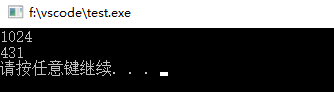注意事项

在做字符串转数字时要注意转换的字符串是否超出了你选用的数据类型的最大值，比如在做"12345678999"转数字时，转换过来的数据类型就不能选用int型，应选用long long型，因为"12345678999"已超出了int所能表达的最大值2147483647。例如下面的代码。

#include <iostream>
#include <string>
#include <sstream>
using namespace std;

int main()
{
string str1("12345678999");
stringstream ss1;
int d1;
ss1 << str1;
ss1 >> d1;
cout << d1 << endl;

stringstream ss2;
long long d2;
ss2 << str1;
ss2 >> d2;
cout << d2 << endl;

system("pause");
return 0;
}

测试结果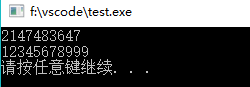2、stoi

stoi定义于头文件<string>中，是C++11标准中新加入的，所以要顺利使用stoi你的编译器必须支持C++11。我们先看stoi在<string>中的定义。

int       stoi( const std::string& str, std::size_t* pos = 0, int base = 10 );
int       stoi( const std::wstring& str, std::size_t* pos = 0, int base = 10 );

第一个参数指定你要转换的字符串，第三个参数指定你要转换的字符串是什么进制的，默认为十进制，第二个参数默认为0，当转换成功时返回int型。类似于stoi转换字符串的函数还有stol、stoul、stoll、stoull、stof、stod、stold，它们的区别只在于转换的字符串类型和返回类型不一样，有兴趣的可以自行了解。

测试代码

#include <iostream>
#include <string>
using namespace std;

int main()
{
string str1("1024");
string str2("1aF");
int d1, d2;
d1 = stoi(str1);
d2 = stoi(str2, 0, 16); //转换的源字符串为十六进制，必须指定第三个参数为16
cout << "stoi(str1):       " << d1 << endl;
cout << "stoi(str2, 0, 16):" << d2 << endl;

system("pause");
return 0;
}

测试结果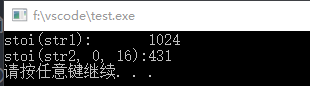注意事项

在使用stoi的时候要注意传入的参数是否有效且转换的结果是否溢出，与stringstream不同，若发生上述错误，stoi直接返回错误代码，程序直接结束运行。所以在使用stoi的时候最好加上异常处理，例如下面的代码。

#include <iostream>
#include <string>
using namespace std;

int main()
{
int d1;
try
{
d1 = stoi("h5hk");
cout << d1 << endl;
}
catch (out_of_range &)
{
cout << "Out of range." << endl;
}
catch (invalid_argument &)
{
cout << "Invalid_argument." << endl;
}
catch (...)
{
cout << "something else wrong." << endl;
}

int d2;
try
{
d2 = stoi("ffffffffff", 0, 16);
cout << d2 << endl;
}
catch (out_of_range &)
{
cout << "Out of range." << endl;
}
catch (invalid_argument &)
{
cout << "Invalid_argument." << endl;
}
catch (...)
{
cout << "something else wrong." << endl;
}

//若转换的数字太大，可以选用处理范围更大的函数，如stoll
long long d3;
try
{
d3 = stoll("ffffffffff", 0, 16);//stoll的返回值为long long型
cout << d3 << endl;
}
catch (out_of_range &)
{
cout << "Out of range." << endl;
}
catch (invalid_argument &)
{
cout << "Invalid_argument." << endl;
}
catch (...)
{
cout << "something else wrong." << endl;
}
system("pause");
return 0;
}

测试结果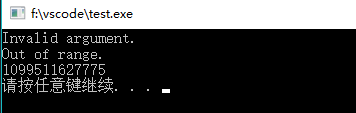3、自己写

如果实在是“看不上”其它方法，那就自己写一个字符串转数字的方法。下面的代码把十六进制字符串转换成int型数字，稍加修改可以转十进制字符串。

#include <iostream>
#include <string>
#include <cmath>
using namespace std;

//十六进制字符转十进制字符
int hextoDec(char c)
{
int ans;
switch (c)
{
case '0':
case '1':
case '2':
case '3':
case '4':
case '5':
case '6':
case '7':
case '8':
case '9':
ans = c - '0';
break;
case 'a':
case 'A':
ans = 10;
break;
case 'b':
case 'B':
ans = 11;
break;
case 'c':
case 'C':
ans = 12;
break;
case 'd':
case 'D':
ans = 13;
break;
case 'e':
case 'E':
ans = 14;
break;
case 'f':
case 'F':
ans = 15;
break;
}
return ans;
}

//字符串str转int型数字
int stringtoInt(string str)
{
int ans = 0;
for (int i = str.size() - 1, j = 0; i >= 0; i--, j++) //从低位到高位依次转化
ans += hextoDec(str[i]) * pow(16, j);             //pow(x, y)返回x^y
return ans;
}

int main()
{
int d = stringtoInt("1aF");
cout << "stringtoInt(\"1aF\"): " << d << endl;
system("pause");
return 0;
}

测试结果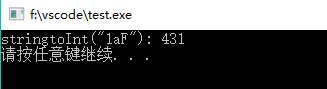展开全文字符串转数字 C++ sstream stoi
• Python3 进制转换(二进制 十进制 十六进制 ASCII字符串转换) Python3 进制转换 编写Convertor类，用于进制与ASCII字符之间互相转换，便于使用 ASCII码及进制之间转换关系 ASCII码介绍： ASCII 全称为 ( American ...
• python 使用递归编写一个十进制转二进制,结果以字符串的形式 网课老师的代码（小甲鱼） def Dect(dec): result='' if dec: result=Dect(dec//2) return result+str(dec%2) else: return result print(Dect(65)...python 字符串
• C语言十进制转二进制代码实例用C语言实现将十进制转化为二进制，并统计转换后的二进制码中1的个数。#include int binaryNum; //存放转换后得到的二进制码int count=0; //计数十进制整数被2除的次数int oneCount=...
• 十进制转二进制. python2,存在int 整型和long(长整型),在python3里就是int/获取的是浮点数 小数 print(bin(15)) 这样可以通过代码来计算十进制数15的二进制十进制数如何转为二进制数:整除法,例如十进制数是15,想...
• 在C语言某个程序当中需要把文本16进制转换成对应的16进制数，比如字符串"0x1a"转换成10进制的26，可以用以下函数来实现相关函数: atof, atoi, atol, strtod, strtoul表头文件: #include 定义函数: long int strtol...
• 返回一个字符串数组，其中每行是S行中每个十进制整数字符串的十六进制表示形式。 S必须是仅包含十进制数字或前导空格的字符数组。 与Matlab不同，此函数可以返回大于2^52整数的精确表示形式。 返回一个字符...
• 先使用strtol函数将字符串转十进制数，再通过sprintf函数将十进制数按十六进制字符串形式输出到字符数组保存 该方案弊端：转换的数不能大于0x7FFFFFFF，因为strtol的返回值是long型 #include <stdio.h> #...
• C语言标准库没有整数转二进制字符串函数，随手写一个：#include "stdio.h"#include #include static const char* LIST = {"0000", "0001", "0010","0011", "0100", "0101", "0110","0111","1000", "1001", "1010...字符串转2进制c语言
• 函数功能：二进制转换成十六进制，与此相反的功能便是十六进制转换成二进制 使用范例： <?php function hex2bin（$data）{$len = strlen($data); for($i = 0; $i <$len; $i +=2) {$newdata ....php 进制转换函数
• 小编典典转换BigInteger为十进制，十六进制，二进制，八进制字符串：让我们从一个BigInteger值开始：BigInteger bigint = BigInteger.Parse("123456789012345678901234567890");基地10和基地16内置的Base 10(十进制)...
• JS十进制转二进制

千次阅读 2020-08-01 08:52:00
主要需求：十进制转二进制，可以控制指定的位数。 转化显示后的二进制数为bin-bit中输入的数字宽度。 dec-number为5，bin-bit为5，则转化后数字为00101。 如果bin-bit小于转化后的二进制本身位数，则使用原本的位数...js
• % SDEC2HEX(D) 返回一个字符串数组，其中每一行是% D 中每个十进制整数的十六进制表示。 % D 必须包含小于 2^52 的有符号整数。 % % SDEC2HEX(D,N) 生成一个字符串数组，其中每个% 行包含一个 N 位十六进制...matlab
• Python字符串与进制转换

千次阅读 2019-09-29 17:49:47
字符 0b / 0B --> 二进制字符前缀 0o / 0O --> 八进制字符前缀 0x / 0X --> 十六进制字符前缀 1、二进制转八进制 数字0和英文b：0b10110011111为二进制数据 ...2、二进制数据转十进制 int函数用于...Python 进制转换 十六进制转字符串 八进制转字符串 十进制转字符串
• //输出 11010 decbin (PHP 3, PHP 4, PHP 5) decbin — 十进制转换为二进制 说明 string decbin ( int number ) 返回字符串，包含有给定 number 参数的二进制表示。所能转换的最大数值为十进制的 4294967295，其...
• //将传入的二进制字符串转换成十进制，并返回十进制数字。 int BinStr2Dec(char const* const binaryString) { //在此处调用判断一个字符串有多长的函数 int size = strlen(binaryString); //将二进制数字...
• 进制转换 /// Converts [this] to a string representation in the given [radix]. /// /// In the string representation, lower-case letters are used for digits above /// '9', with 'a' being 10 an 'z' being...dart flutter string 二进制转换 十六进制转换javascript
• @param $binary_string 参数表示所要转换的二进制字符串。 @return 返回与$binary_string参数所表示的二进制数的十进制数等价值。 函数说明： bindec()将一个二进制数转换成Integer类型或者出于大小的需要，转换为...
• } View Code 以上是10进制16进制的代码，可以调用int StrDec2HexBuf( const StrVal& strDec, BYTE* destBuf )，将strDec的十进制数据转换成16进制的destBuf字符串，并返回destBuf的有效长度 关于10进制16进制的...
• 先上用Python写的十进制转二进制的函数代码：def Dec2Bin(dec):result = ''if dec:result = Dec2Bin(dec//2)return result + str(dec%2)else:return resultprint(Dec2Bin(62))图解此函数执行过程：文字描述此函数的...
• B返回值：当A、B中元素均为非零元素是，返回1，否则，为0，还可表示成and(a,b);|：逻辑或A|B。还可以表示成or(A,B).~ : 逻辑非 ~A。还可以表示成not(A).xor:逻辑异或xor(A,B)./////////////////////////////////////...
• 目录一、C/C++标准库执行1、字符串转数字2、数字格式化为字符串、非标实现1、十进制数字字符串转数值2、十六进制数字字符串转数值3、、八、十进制间转换，以十进制方式显示4、整形数字转字符串5、浮点型数字转...c/c++ 进制转换 字符串 大小端 标准库
• 展开全部toHexStringpublic static String toHexString(inti)以十六进制的无符号整数形式返回一个整数参数的字符串表示形式。如果参数为负，那么无符号整数值为参62616964757a686964616fe4b893e5b19e31333339666132...
• 来了老弟，emmmmm,今天想到平时经常用到编码转化，把字符串转化为16进制绕过等等的，今天想着用python写个玩，查询了一些资料，看了些bolg 上面的两个函数是将二进制流转化为16进制，data的每一个比特都被转为...
• 首页>基础教程>常用类>常用 Integer类Java Integer....定义String toOctalString(int i) //以八进制(基数 8)无符号整数形式返回一个整数参数的字符串表示形式。也就是将传入的数值十进制转成八进制......